Test: Construction of Similar Figures

# Test: Construction of Similar Figures

Test Description

## 15 Questions MCQ Test NCERT Mathematics for CAT Preparation | Test: Construction of Similar Figures

Test: Construction of Similar Figures for Class 10 2022 is part of NCERT Mathematics for CAT Preparation preparation. The Test: Construction of Similar Figures questions and answers have been prepared according to the Class 10 exam syllabus.The Test: Construction of Similar Figures MCQs are made for Class 10 2022 Exam. Find important definitions, questions, notes, meanings, examples, exercises, MCQs and online tests for Test: Construction of Similar Figures below.
Solutions of Test: Construction of Similar Figures questions in English are available as part of our NCERT Mathematics for CAT Preparation for Class 10 & Test: Construction of Similar Figures solutions in Hindi for NCERT Mathematics for CAT Preparation course. Download more important topics, notes, lectures and mock test series for Class 10 Exam by signing up for free. Attempt Test: Construction of Similar Figures | 15 questions in 15 minutes | Mock test for Class 10 preparation | Free important questions MCQ to study NCERT Mathematics for CAT Preparation for Class 10 Exam | Download free PDF with solutions
 1 Crore+ students have signed up on EduRev. Have you?
Test: Construction of Similar Figures - Question 1

### In the figure, DE is parallel to BC such that AE = one fourth of AC. If AB = 6cm, then AD is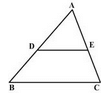​

Detailed Solution for Test: Construction of Similar Figures - Question 1 Let AC be x. then *(1÷4) + (3÷4)=x AC =1Now In ∆ABC . (by BPL)AD/AB = AE/ACAD=1/4×6AD=1.5 cm
Test: Construction of Similar Figures - Question 2

### Which of the following relation would hold true for the sides of the similar triangles in the given diagram?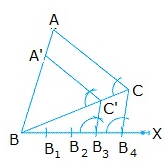Test: Construction of Similar Figures - Question 3

### The perimeters of two similar triangles are 25 cm and 15 cm respectively. If one side of first triangle is 9 cm, the corresponding side of the other triangle is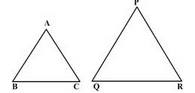​

Detailed Solution for Test: Construction of Similar Figures - Question 3

In similar triangles, the perimeter of the triangles will be in the ratio of their corresponding sides.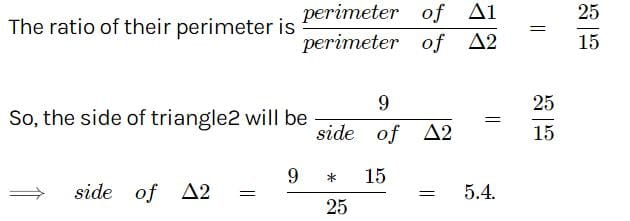Test: Construction of Similar Figures - Question 4

In the given figure, AC: CB is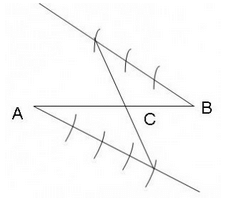Test: Construction of Similar Figures - Question 5

In the figure QA and PB are perpendiculars on AB. If AO = 10 cm, BO = 6 cm and PB = 9cm, then AQ is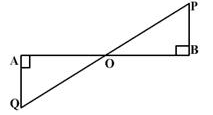Test: Construction of Similar Figures - Question 6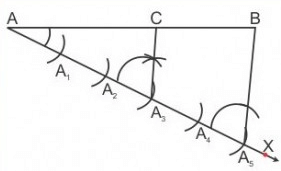From the given figure, find out what is AC : AB.​

Test: Construction of Similar Figures - Question 7

Sides of two similar triangles are in the ratio 3:8. Areas of these triangles are in the ratio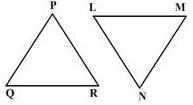Test: Construction of Similar Figures - Question 8

In the given figure, triangles ABC and DEF are similar. The area of triangle ABC is 9 sq. cm and the area of triangle DEF is 16 sq. cm. If BC = 2.1 cm find the length of EF.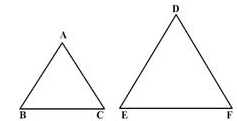Test: Construction of Similar Figures - Question 9

In the given figure, triangle ABC has been constructed similar to triangle A’BC’. What is the scale factor?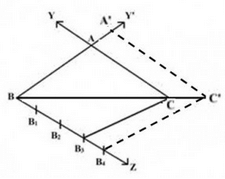Test: Construction of Similar Figures - Question 10

In the figure, DE is parallel to BC such that AE = one fourth of AC. If AB = 6cm, then AD is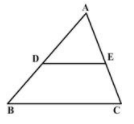Test: Construction of Similar Figures - Question 11

In drawing triangle ABC, it is given that AB = 3 cm, BC = 2 cm and AC = 6 cm. It is not possible to draw the triangle as:​

Test: Construction of Similar Figures - Question 12

Identify the incorrect statement.​

Test: Construction of Similar Figures - Question 13

ABCD is a quadrilateral with BC = 8 cm. Quadrilateral AB’C’D’ is constructed with a scale factor 3/4. What will be the length of B’C’?​

Detailed Solution for Test: Construction of Similar Figures - Question 13

The quadrilateral is dilated by a scale factor of 3/4.The shape of image will be same as pre image but size will vary means it will be smaller .If Dilation factor is , means 0< Dilation factor<1, the image is be smaller than preimage.
So new length =k*original size=¾*8 = 6cm
So B’C’ = 6cm

Test: Construction of Similar Figures - Question 14

In the given figure, AB and DE are perpendiculars to BC. If AB = 9cm, DE = 3cm and AC = 24cm then AD is equal to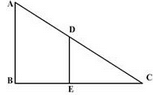Detailed Solution for Test: Construction of Similar Figures - Question 14

Since AB||DE so Angle A= Angle D,Angle C is common
So by AA criterion, ΔABC - ΔDEC
So,the corresponding sides are in proportion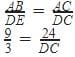DC=8

Test: Construction of Similar Figures - Question 15

If triangles PQR and DEF are similar, then which of the similarity criterion is true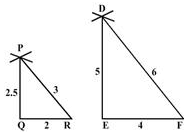## NCERT Mathematics for CAT Preparation

276 docs|149 tests
 Use Code STAYHOME200 and get INR 200 additional OFF Use Coupon Code
Information about Test: Construction of Similar Figures Page
In this test you can find the Exam questions for Test: Construction of Similar Figures solved & explained in the simplest way possible. Besides giving Questions and answers for Test: Construction of Similar Figures, EduRev gives you an ample number of Online tests for practice

## NCERT Mathematics for CAT Preparation

276 docs|149 tests